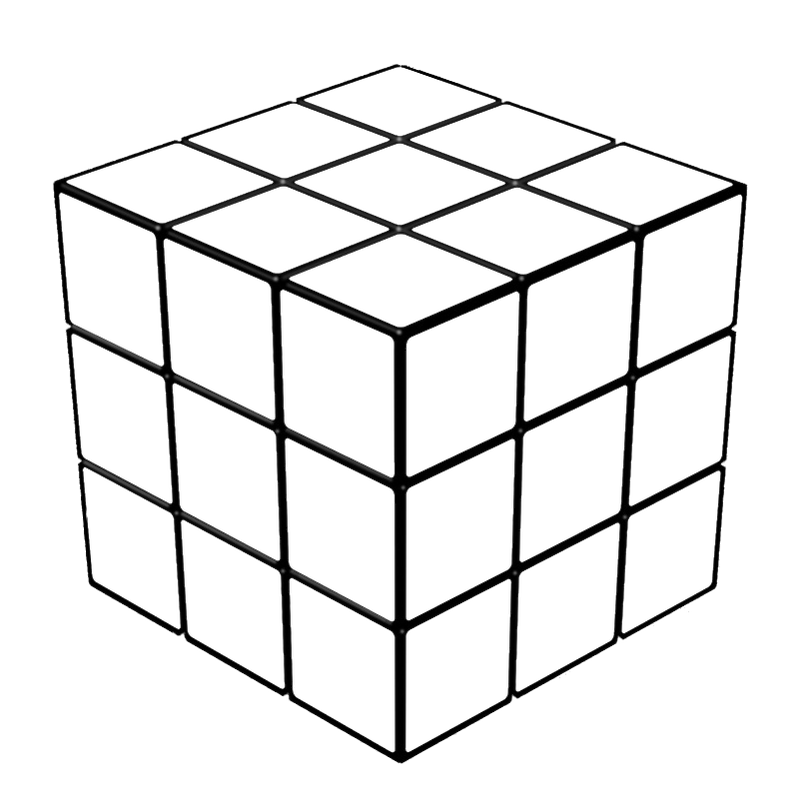# Maximizing Surface Area

Directions: The following prism is made up of 27 identical cubes. What is the greatest possible surface area the prism can have after removing 1 or more cubes from the outside?### Hint

How many cubes (and which ones) would you remove? How could you record the information to help you see a pattern?

Removing the center cube in each face of the prism results in a surface area of 78 square units.

Source: Brian Lack

## Greatest Common Factor

Directions: Using the digits 0 to 9 at most one time each, place a digit …

1.That’s a cool problem, but I don’t understand the title. Isn’t the point NOT to keep the surface area constant? Puzzled…

•Robert Kaplinsky

Darn good point Julie, as always. I’ll change the title.

2.A colleague has found an additional solution with a surface area of 78 square units:
Remove four cubes on each face – the center cube on the top and bottom row; the two cubes on the ends of the middle row.
This additional solution prompted conversations about the comparison of surface area to volume, as well as real-world instances where maximizing (or minimizing) the ratio of surface area to volume is important.

3.Could a student think that the phrase “removing cubes from the outside” means removing a layer therefore the student would remove 9 cubes.

•I tweaked the language a bit to say “removing 1 or more cubes from the outside.” Does that tighten it up a bit?

•Weird how the title says maximizing when your not supposed to change the surface area…

•Hi Cameron. What makes you think that is says that you’re not supposed to change the surface area?

4.Can you remove the X on all sides and still get 78? So the middle and all corners are gone. I believe it is the same solution and could set up a greatest number of blocks you can remove and still have the largest surface area possible.

5.i dont understand how you got 78

•can you help me understand

•Imagine one face of the cube, not all six faces. If you remove the center cube from one of the faces, the surface area for that face is 13 square units. You multiply 13 by 6, then you will get 78 square units.

6.If you multiply 13by 6 you will get78 square units.

7.Hello,
I am very confused why one would do 13 X 6 to get 78 square units as the answer for this problem. First of all, doesn’t the surface area start out as 9 X 6 (meaning 54 square units) BEFORE removing one cube from each of the 6 faces? So how does surface area go from 9 X 6 to 13 X 6 when you’re removing cubes? Maybe I’m having a hard time with redistributing volume/surface area after cube removal so maybe some one could tell me how many layers the prism ends up having after removing cubes and rearranging….
I wish I had my connecting cubes at home 😛

•I now understand where ’13’ on a face comes from, but wouldn’t there only be 2 layers of 13 (volume of 26) to make the figure, assuming you’ve only removed one cube? If the figure had 6 sides with surface area of 13 for each (13 X 6) like mentioned above, that would make a volume much greater than the starting volume of 27, even though we’re technically ‘removing’ at least 1 cube…..

Thanks for anyone’s help on this!

•Got it everyone!!! I actually found a highest surface area answer of 82 with this instead of 78….all my previous comments got my brain rolling. Here’s what I found:

If you were to take just ONE cube away from the original prism, that leaves a volume of 26 cubic units to play with. If you were to rearrange those 26 cubic units into two layers/rows of 13 cubes, you would technically have two faces (the ‘one’ row showing sides) that each have a surface area of 13, two sides that have a surface area of 26 (because of your two faces that show 2 rows of 13 units side by side), and then your ‘ends’ would have a surface area of 2 each (the ‘ends’ of your two rows of 13). So the expressions representing the 6 faces would be as follows: (2 faces * 13 units) + (2 faces * 26 units) + (2 faces * 2 units)= 82

Let me know if I made an error in that, but I think that would be right.